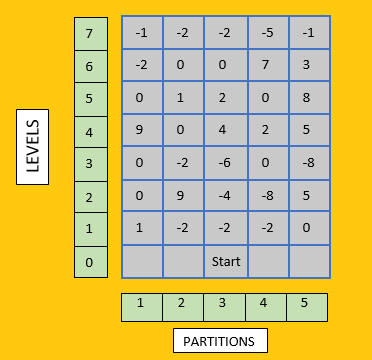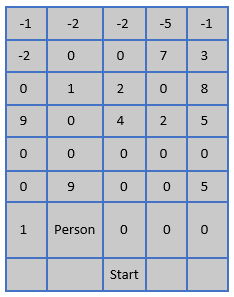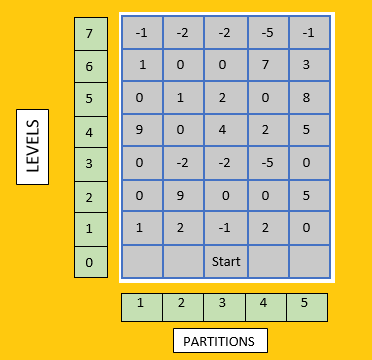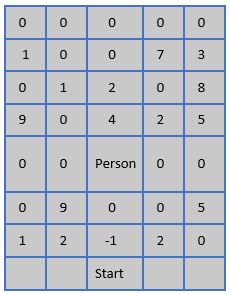Owl and Stairs
/

## Dynamic Programming

Problem
Editorial
Analytics

There is a staircase consisting of $N + 1$ levels (0 to N). Each step contains M(1 to M) partitions. This can be treated as a $(N + 1)$ x M matrix. An owl wishes to cross all the levels.

Now, a cell of the matrix may be empty, contain a health medicine, or contain a thorn. If he steps on a thorn, his health decreases by that value. If he steps on a health medicine, his health increases by that value. If he steps on an empty cell, nothing happens.

To help him, he has a friend who will remove all the thorns present up to M levels up from the current level. For example, if he is currently on level 2 (and M = 3) and he calls his friend, his friend will remove all the thorns present at the levels 3, 4 and 5. He can call his friend only $once$.

He is initially at health 0 and if at any level his health becomes less than 0, he dies.

He is initially standing at the 0$th$ level at the K$th$ partition ( 1 $<=$ K $<=$ M ). The 0th level is empty.

If he is currently at i$th$ level and j$th$ partition, then he has these choices:

• Move to the $j+1$$th$ partition of $i+1$$th$ level.
• Move to the j$th$ partition of the $i+1$$th$ level.
• Move to the $j-1$$th$ partition of the $i+1$$th$ level.
• If he hasn’t called his friend, he can call him to remove the thorns present on levels i+1,i+2, … , i+M. and move to any one of the above three choices.

Thorns are represented by a value less than zero. Health medicine is represented by a value greater than zero and 0 is an empty cell. The 0th level has all 0’s.

He wants to know the maximum health with which he can cross the Nth level. He is very scared and needs your help. If he cannot cross the Nth level, that is, there is no way in which he can reach alive print 1 .

Constraints:
1 $<=$ T $<=$ $25$
1 $<=$ $N,M$ $<=$ $100$
1 $<=$ K $<=$ M
M $<=$ N
$-200$ $<=$ Elements of the matrix $<=$ $200$

Input Format
First line will contain number of test cases T.
First line of each test case will contain 3 integers N,M and K, denoting the number of levels, number of partitions and the start partition respectively.
Next N lines will contain M integers.

Output Format
Output a single integer denoting the maximum health with which the person can cross the N$th$ level. Or output 1 if there is no way in which he can cross the N$th$ level alive.

Problem Setter - Harsh Jain

SAMPLE INPUT
4
7 5 3
-1 -2 -2 -5 -1
-2 0 0 7 3
0 1 2 0 8
9 0 4 2 5
0 -2 -6 0 -8
0 9 -4 -8 5
1 -2 -2 -2 0
7 5 3
-1 -2 -2 -5 -1
1 0 0 7 3
0 1 2 0 8
9 0 4 2 5
0 -2 -2 -5 0
0 9 0 0 5
1 2 -1 2 0
4 3 2
-1 -1 -1
0 0 0
1 1 1
-1 -1 -1
4 3 2
-1 -1 -1
0 0 0
0 0 0
-1 -1 -1
SAMPLE OUTPUT
25
28
0
-1
Explanation

The sample case 1 can be seen as this:Step 1: In this, he cannot move to level 1 as his life will become less than 0. So, he calls his friend and destroys all the thorns on levels 1,2,3,4 and 5 (M = 5). Now he will move to (1,2). [Level - 1, Partition - 2]

Note: He has to call his friend and move to the next level in the same step.

The matrix looks like this now.Step 2: He will move to (2,2) and gain 9 health.
Step 3: He will move to (3,3).
Step 4: He will move to (4,4) and gain 2 health.
Step 5: He will move to (5,5) and gain 8 health.
Step 6: He will move to (6,4) and gain 7 health.
Step 7: He will move to (7,5) and loose 1 health.

Total: 9 + 2 + 8 + 7 – 1 = 25

The sample case 2 can be seen as this.Step 1: He will move to (1,2) and gain 2 health.
Step 2: He will move to (2,2) and gain 9 health.
Step 3: He will call his friend to remove the thorns and move to (3,3) and now there is no thorn on (3,3) so gains 0 health.

The matrix now looks like this.Step 4: He will move to (4,4) and gain 2 health.
Step 5: He will move to (5,5) and gain 8 health.
Step 6: He will move to (6,4) and gain 7 health.
Step 7: He will move to (7,4).

Total: 2 + 9 + 2 + 8 + 7 = 28

Time Limit: 1.0 sec(s) for each input file.
Memory Limit: 256 MB
Source Limit: 1024 KB

## This Problem was Asked in

Initializing Code Editor...